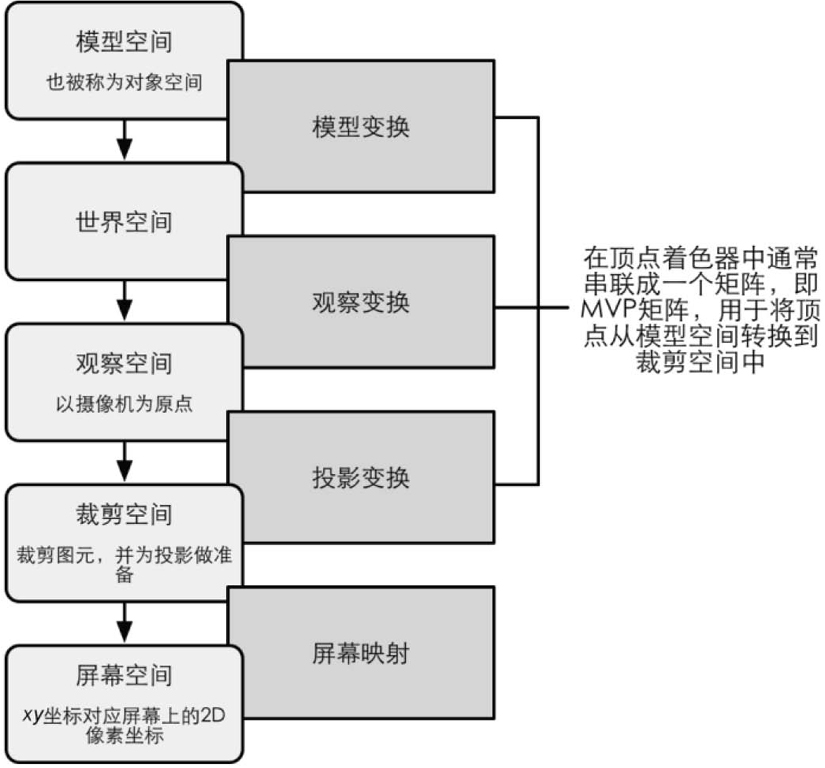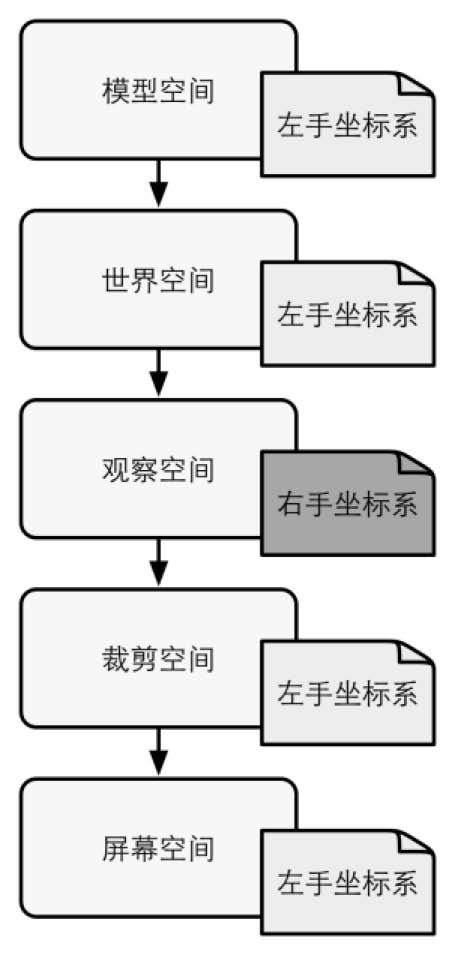/  /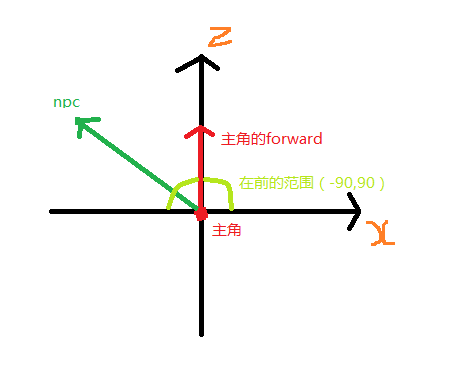```Transform targetNpc;
void Start () {
targetNpc = GameObject.Find("Npc").transform;
//计算在前还是在后  cos [-90,90] 为 [0,1]
float res = Vector3.Dot(transform.forward, targetNpc.position - transform.position);
if (res > 0)
{
Debug.Log("在前面");
}
else if (res < 0)
{
Debug.Log("在后面");
}
else
{
Debug.Log("在正左或右");
}
}```

```void Start () {
Vector3 v = Vector3.Cross(target.position - transform.position，transform.forward);
Debug.Log(v.y);//正在左，负在右
}```

```using UnityEngine;
public class TestWXS : MonoBehaviour {

float x = 0;
float z = 0;

void Update () {
Resetpos();
//x++;
z++;
transform.Rotate(0, 0, z);
transform.Rotate(0, 90, 0);
transform.Rotate(x, 0, 0);
}

private void Resetpos()
{
transform.position = Vector3.zero;
transform.rotation = Quaternion.identity;
}
}```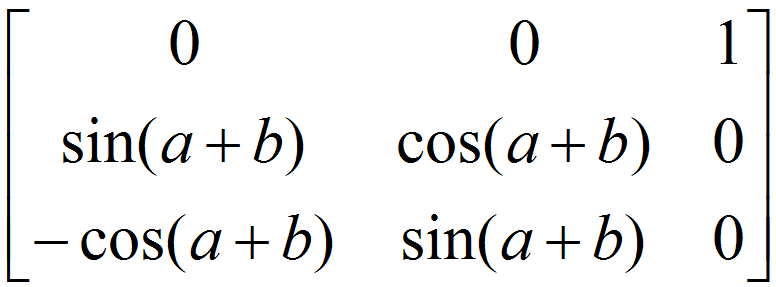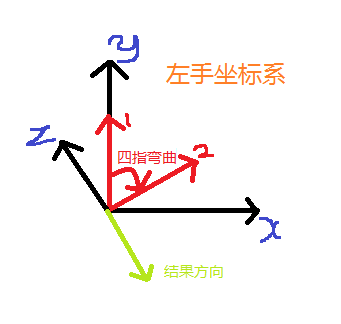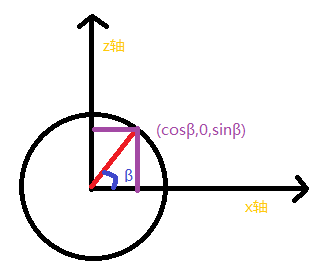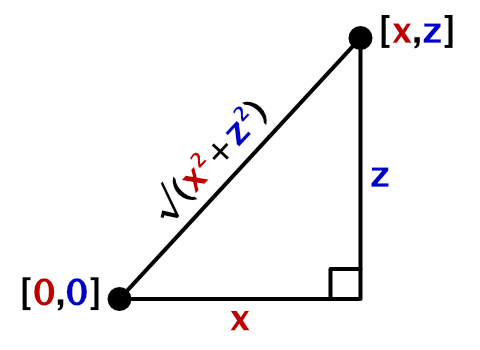distance = sqrt(x2 + y2 + z2)